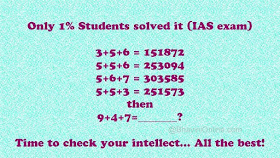## Saturday, 28 August 2021

### MATHS PUZZLE NO-3 WITH ANSWER

Brain Math Puzzle is a game to improver your Logical Skills & Reasoning.

Brain Math Puzzle is a game to improver your Logical Skills & If you like mathematics than this game is made for you.

More than 150 levels in game.

More than 20 different Objects used in a game.

The solution to the first 4 digits is pretty straight forward, and I am sure most of you must have easily got it.deal.Only 1% Students solved it (IAS exam)

3+5+6=151872

5+5+6=253094

5+6+7=303585

5+5+3=251573

then 9+4+7=_______?

It works out to be a+b+c = (a*b)(a*c)??

The last 2 digits are really tough to crack…

Here’s how you do it. Reverse of {(a×b)+(a×c)-c}

So the number would (a×b),(a×c),{(a×b)+(a×c)-c}reverse.

So for 1st one: 3+5+6=151872;
axb = 3×5 = 15; axc = 3×6 = 18;
{(a×b)+(a×c)-c} = ((3×5)+(3×6)-6} = 27
— Reverse of 27 is 72

So 9+4+7=_______?

axb = 9×4 = 36;
axc = 9×7 = 63;
{(a×b)+(a×c)-c} = ((9×4)+(9×7)-7} = (36+63-7)=92
— Reverse of 92 is 29.

Answer :::: Hence 9+4+7 = 366329

Math puzzle

Simple math puzzle game.

More than 200 different levels.

Take a block from panel and make equation.

Use hints when you getting stuck.

Easy to play hard to master.

How to Play ?

Solve the equation & Give the answer.

For Each game start you will get 3 life.So if you wrong some where you will get another chance.

No time limits.

Who can play ?

Every math lover can play this game no age limit.

If u r genius solve it:-

40 × 14 = 11

30 × 13 = 12

20 × 12 = 6

10 × 11 = ?

Logic :

40×14 = 560 = 5+6+0 = 11

30×13 = 390 = 3+9+0 = 12

20×12 = 240 = 2+4+0 = 6

So 10×11 =110 = 1+1+0 = 2

Game Features

•Realistic graphics and ambient sound.

•Realistic 2D stunning and amazing animations.

•Smooth and simple controls.

•User friendly interface and interactive graphics.

Important Point

Use BODMAS Rules & keep Focus on Objects.

Benefits

improves mathematics skills.

Improves logical skill.

Improve reasoning.